# 低成本红外热成像系统研制Development of Low Cost Infrared Thermal Imaging System

• 全文下载: PDF(1589KB)    PP.74-84   DOI: 10.12677/JISP.2018.72009
• 下载量: 637  浏览量: 1,663   科研立项经费支持

This paper presents a low-cost infrared thermal camera image processing system. The program takes the ZYNQ-7000 series SoC development board produced by Xilinx as the core processing element, whereby the Raspberry Pi is used as an adapter for the far-infrared camera and the FILR Lepton LWIR is selected as the image acquisition device. After the LWIR gets the image, it passes the Raspberry Pi through the SPI interface, and then uses the TCP socket to send the image to the SoC FPGA for processing. By highlighting the parallelism of the FPGA features, the original image can be quickly improved algorithm image. This paper, taking image super-resolution reconstruction and real-time CANNY operator as an example, conducted experiments, which show that the system has the characteristics of low cost, low power consumption and ease of use.

1. 引言

2. 系统设计

2.1. 图像采集模块

FLIR Lepton LWIR传感器的结构图如图2所示。

FLIR Lepton LWIR相机的镜头将红外线聚焦到80 × 60的热探测器阵列上，每个探测器单元是一个氧化钒微测热辐射计，其温度的变化受入射光通量的影响。每个微测热辐射计的电阻变化量与温度的变Table 1. FLIR Lepton LWIR main parameters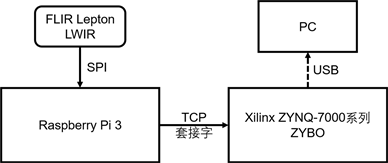Figure 1. Far-infrared image processing system design diagram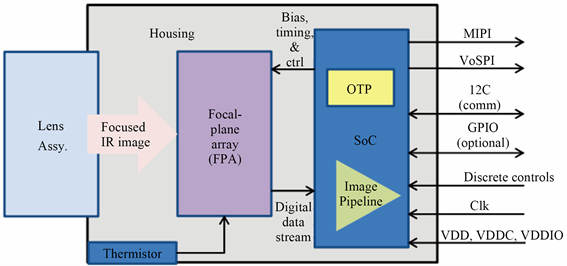Figure 2. FLIR Lepton LWIR Sensor Block Diagram

FLIR Lepton LWIR提供VoSPI协议数据接口，我们通过SPI端口将其与Raspberry Pi 3相连。连接方式如图3所示。

FLIR Lepton LWIR使用SPI模式3 (CPOL = 1，CPHA = 1)，空闲时SCK为高电平。数据由FLIR Lepton LWIR在SCK的下降沿建立，并由主控制器在上升沿采样。数据首先以高位顺序传送最高有效字节。时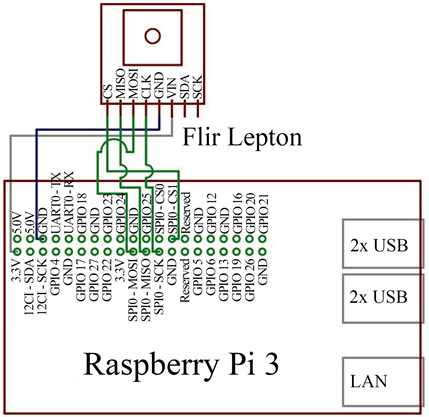Figure 3. Schematic diagram of FLIR Lepton LWIR connection to Raspberry Pi 3

2.2. 图像传输模块

Raspberry Pi 3与ZYBO开发板之间的通信使用的是TCP套接字，通过LAN实现红外图像的稳定传输。

Raspberry Pi 3在系统中作为“SPI转LAN”的适配器，在网络传输时作为服务器发送采集的红外图像数据。上电后其运行的服务器软件的主要流程为：

1) 打开SPI端口、打开并绑定套接字，让客户端能连接服务器。

2) 在初始化套接字后，等待客户端的请求并连接客户端。

3) 建立与客户端的连接后，接收SPI传输的红外图像数据。

4) 将采集的图像数据分割为4份缓冲区，每份1200字节。每次传输15行的图像数据，为防止冻结或错误，我们检查是否所有的数据包都被发送，否则将关闭软件，结束传输，等待重新传输。

2.3. 图像处理模块

1) 超分辨率模型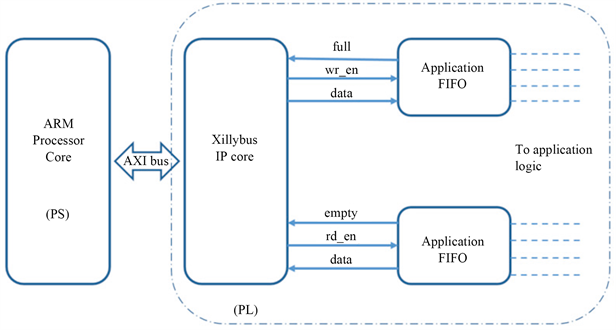Figure 4. ZYBO internal logic diagram

$\left\{{V}_{k}|k=1,2,3,\cdots ,N\right\}$ (1)

$L={I}_{h}×{I}_{v}$ (2)

$H={r}_{h}{I}_{h}×{r}_{v}{I}_{v}$ (3)

${V}_{k}={D}_{k}{B}_{k}{H}_{k}I+{\eta }_{k},\text{\hspace{0.17em}}\text{\hspace{0.17em}}k=1,2,3,\cdots ,N$ (4)

2) 压缩感知理论

$\Psi =\left[{\phi }_{1},{\phi }_{2},\cdots ,{\phi }_{N}\right]$ (5)

${R}^{N}$ 空间中的任意信号x都可以由基向量 ${\phi }_{i}$ 或基矩阵Ψ表示为：

$x={\sum }_{i=1}^{N}{\alpha }_{i}{\phi }_{i}$$x=\Psi \alpha$ (6)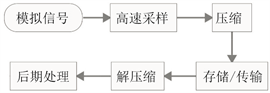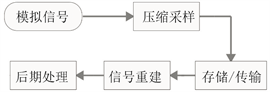Figure 6. Compressed sensing sampling flowchart

3) 图像超分辨重建

$Y=BDX$ (7)

$\chi \approx {D}_{h}\alpha$$\alpha \in {ℝ}^{K}$${‖\alpha ‖}_{0}\ll K$ (8)

4) 重建流程

3. 实验

Canny边缘检测算子是John F. Canny于1986年开发出来的一个多级边缘检测算法，自问世以来，Canny边缘检测算子一直是边缘检测的经典算法。Canny算子的基本思想是：先对处理的图像选择一定的Gauss滤波器进行平滑滤波，然后采用一种称之为“非极值抑制”(Non-maximum Suppression)的技术，对平滑后的图像处理后，得到最后所需的边缘图像  。

4. 结论

FLIR Lepton LWIR使用功耗约为0.15 W，Raspberry Pi 3约为0.65 W，ZYBO约为1 W，总计约1.8 W。在功耗方面无法直接进行比较，但是横向对比FLIR公司另一款产品Vue Pro无人机用红外热成像仪，Figure 7. Original image of the portrait taken by FLIR Lepton LWIR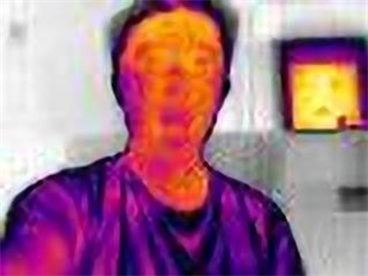Figure 8. The portrait taken by FLIR Lepton LWIR after 4 times reconstructionFigure 9. Original Raspberry Pi 3 image taken by FLIR Lepton LWIR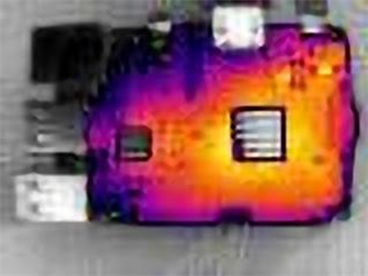Figure 10. Raspberry Pi 3 image taken by FLIR Lepton LWIR after 4 times reconstruction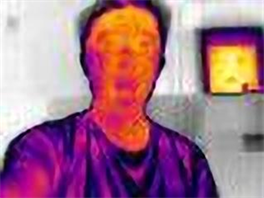Figure 11. Portrait image (left) and 4× reconstruction (right)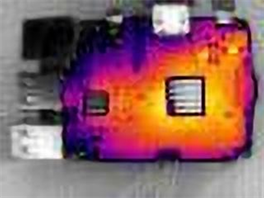Figure 12. Raspberry Pi 3 image original (left) and after 4 times reconstruction (right)Figure 13. Portrait image (left) and after Canny operation (right)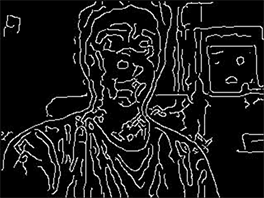Figure 14. Portrait image after 4 times reconstruction (left) and after Canny operation (right)Figure 15. Raspberry Pi 3 image (left) and after Canny operation (right)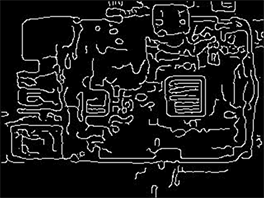Figure16. Raspberry Pi 3 image after 4 times reconstruction (left) and Canny operation (right)

NOTES

*通讯作者。

  孙志君. 红外焦平面阵列技术的未来二十年[J]. 传感器世界, 2002, 8(11): 1-8.  彭焕良. 热成像技术发展综述[J]. 激光与红外, 1997, 27(3): 131-136.  章睿. 便携式非制冷红外成像设备的设计[J]. 国外电子测量技术, 2007, 26(4): 65-67.  高翔宇. 基于Linux操作系统下的TCP/IP网络通信研究与应用[J]. 现代电子技术, 2006, 233(18): 74-76.  花歆悦. 基于压缩感知的声纳图像超分辨率重建算法[J]. 微型机与应用, 2015, 34(13): 49-52.  Ng, M.K. and Bose, N.K. (2003) Mathematical Analysis of Super-Resolution Methodology. IEEE Signal Processing Magazine, 20, 62-74. https://doi.org /10.1109/MSP.2003.1203210  Candès, E., Romberg, J. and Tao, T. (2006) Robust Uncertainty Principles: Exact Signal Re-construction from highly Incomplete Frequency Information. IEEE Transactions on Information Theory, 52, 489-509. https://doi.org /10.1109/TIT.2005.862083  Donoho, D.L. (2006) Compressed Sensing. IEEE Transactions on In-formation Theory, 52, 1289-1306. https://doi.org /10.1109/TIT.2006.871582  刘娟. 基于压缩感知的单幅图像超分辨率重建算法研究[D]: [硕士学位论文]. 成都: 电子科技大学, 2012.  Yang, J., Wright, J., Huang, T. and Ma, Y. (2008) Image Super-Resolution as Sparse Representation of Raw Image Patches. IEEE Conference on Computer Vision and Pattern Recognition, Anchorage, AK, 23-28 June 2008, 1-8.  林卉, 赵长胜, 舒宁. 基于Canny算子的边缘检测及评价[J]. 黑龙江工程学院学报, 2003, 17(2): 3-6.